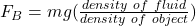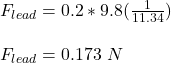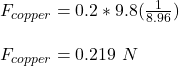## A solid 200-g block of lead and a solid 200-g block of copper are completely submerged in an aquarium filled with water. Each block is suspe

Question

A solid 200-g block of lead and a solid 200-g block of copper are completely submerged in an aquarium filled with water. Each block is suspended just above the bottom of the aquarium by a thread. Which of the following is true?
B. The buoyant force on the copper block is greater than the buoyant force on the lead block.
C. The buoyant force on the lead block is greater than the buoyant force on the copper block.
D. The buoyant force is the same on both blocks.

in progress 0
1 month 2021-08-13T19:43:10+00:00 1 Answers 1 views 0

B. The buoyant force on the copper block is greater than the buoyant force on the lead block.

Explanation:

Given;

mass of lead block, m₁ = 200 g = 0.2 kg

mass of copper block, m₂ = 200 g = 0.2 kg

density of water, ρ = 1 g/cm³

density of lead block, ρ₁ = 11.34 g/cm³

density of copper block, ρ₂ = 8.96 g/cm³

The buoyant force on each block is calculated as;The buoyant force of lead block;The buoyant force of copper blockTherefore, the buoyant force on the copper block is greater than the buoyant force on the lead block